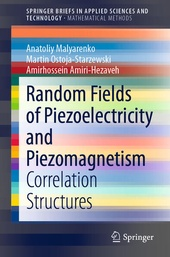# Random Fields of Piezoelectricity and Piezomagnetism

Correlation Structures

Springer (Verlag)
• erscheint ca. am 29. November 2020

• Buch
• |
• Softcover
• |
• X, 91 Seiten
978-3-030-60063-1 (ISBN)

Random fields are a necessity when formulating stochastic continuum theories. In this book, a theory of random piezoelectric and piezomagnetic materials is developed. First, elements of the continuum mechanics of electromagnetic solids are presented. Then the relevant linear governing equations are introduced, written in terms of either a displacement approach or a stress approach, along with linear variational principles. On this basis, a statistical description of second-order (statistically) homogeneous and isotropic rank-3 tensor-valued random fields is given. With a group-theoretic foundation, correlation functions and their spectral counterparts are obtained in terms of stochastic integrals with respect to certain random measures for the fields that belong to orthotropic, tetragonal, and cubic crystal systems. The target audience will primarily comprise researchers and graduate students in theoretical mechanics, statistical physics, and probability.

 Reihe: Auflage: 1st ed. 2020 Sprache: Englisch Verlagsort: Cham | Schweiz Verlagsgruppe: Springer International Publishing Zielgruppe: Für Beruf und Forschung Illustrationen: 1 | 1 farbige Abbildung | Approx. 100 p. Maße: Höhe: 23.5 cm | Breite: 15.5 cm Schlagworte: ISBN-13: 978-3-030-60063-1 (9783030600631) DOI: 10.1007/978-3-030-60064-8weitere Ausgaben werden ermittelt

Preface.- 1. Continuum Theory of Piezoelectricity and Piezomagnetism.- 2. Mathematical preliminaries.- 3. The Choice of a Basis in the Space VG.- 4. Correlation Structures.- References.- Index.

Schweitzer Klassifikation
Thema Klassifikation
Newbooks Subjects & Qualifier
• Mathematik | Informatik
•Mathematik
•Stochastik
•Stochastische Prozesse
• Mathematik | Informatik
•Mathematik
•Stochastik
•Wahrscheinlichkeitsrechnung
• Naturwissenschaften
•Physik
•Elektromagnetismus
•Magnetismus
• Naturwissenschaften
•Physik
•Mechanik
•Kontinuumsmechanik, Strömungslehre
• Naturwissenschaften
•Physik
•Thermodynamik
•Festkörperphysik, Kondensierte Materie
DNB DDC Sachgruppen
Dewey Decimal Classfication (DDC)
• Science
•Mathematics
•Mathematics
• Science
•Mathematics
•Probabilities & applied mathematics
• Science
•Physics
•Classical mechanics
• Science
•Physics
•Magnetism
• Science
•Physics
•Physics
BIC Classifikation
• Mathematics & science
•Mathematics
•Applied mathematics
•Stochastics
• Mathematics & science
•Mathematics
•Probability & statistics
• Mathematics & science
•Physics
•Classical mechanics
•Fluid mechanics
BISAC Classifikation
• Science
•Mechanics / General
• Science
•Physics / General
•Condensed Matter
• Science
•Physics / General
•Magnetism
Warengruppensystematik 2.0
 Ein kurzer Link zu dieser Seite Kopieren Sie diesen Link, um ihn an Dritte weiterzuleiten:als Link merkennach obenDrucken

Noch nicht erschienen

 53,49 € inkl. 5% MwSt.